Search by Topic

Resources tagged with Working systematically similar to Ladybird Count:

Filter by: Content type:
Age range:
Challenge level:

There are 321 results

Broad Topics > Using, Applying and Reasoning about Mathematics > Working systematicallyPage Numbers

Age 7 to 11 Short Challenge Level:

Exactly 195 digits have been used to number the pages in a book. How many pages does the book have?Code Breaker

Age 7 to 11 Challenge Level:

This problem is based on a code using two different prime numbers less than 10. You'll need to multiply them together and shift the alphabet forwards by the result. Can you decipher the code?Number Sandwiches

Age 7 to 14 Challenge Level:

Can you arrange the digits 1, 1, 2, 2, 3 and 3 to make a Number Sandwich?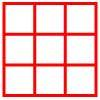Age 5 to 11 Challenge Level:

Place six toy ladybirds into the box so that there are two ladybirds in every column and every row.Junior Frogs

Age 5 to 11 Challenge Level:

Have a go at this well-known challenge. Can you swap the frogs and toads in as few slides and jumps as possible?Coded Hundred Square

Age 7 to 11 Challenge Level:

This 100 square jigsaw is written in code. It starts with 1 and ends with 100. Can you build it up?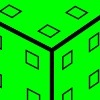Inky Cube

Age 7 to 14 Challenge Level:

This cube has ink on each face which leaves marks on paper as it is rolled. Can you work out what is on each face and the route it has taken?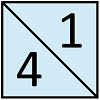Jumping Squares

Age 5 to 7 Challenge Level:

In this problem it is not the squares that jump, you do the jumping! The idea is to go round the track in as few jumps as possible.First Connect Three

Age 7 to 14 Challenge Level:

Add or subtract the two numbers on the spinners and try to complete a row of three. Are there some numbers that are good to aim for?Mrs Beeswax

Age 5 to 7 Challenge Level:

In how many ways could Mrs Beeswax put ten coins into her three puddings so that each pudding ended up with at least two coins?A Square of Numbers

Age 7 to 11 Challenge Level:

Can you put the numbers 1 to 8 into the circles so that the four calculations are correct?Difference

Age 7 to 11 Challenge Level:

Place the numbers 1 to 10 in the circles so that each number is the difference between the two numbers just below it.Find the Difference

Age 5 to 7 Challenge Level:

Place the numbers 1 to 6 in the circles so that each number is the difference between the two numbers just below it.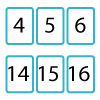Twenty Divided Into Six

Age 7 to 11 Challenge Level:

Katie had a pack of 20 cards numbered from 1 to 20. She arranged the cards into 6 unequal piles where each pile added to the same total. What was the total and how could this be done?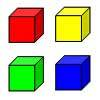Four Colours

Age 5 to 11 Challenge Level:

Kate has eight multilink cubes. She has two red ones, two yellow, two green and two blue. She wants to fit them together to make a cube so that each colour shows on each face just once.Spell by Numbers

Age 7 to 11 Challenge Level:

Can you substitute numbers for the letters in these sums?Dice Stairs

Age 7 to 11 Challenge Level:

Can you make dice stairs using the rules stated? How do you know you have all the possible stairs?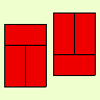Fault-free Rectangles

Age 7 to 11 Challenge Level:

Find out what a "fault-free" rectangle is and try to make some of your own.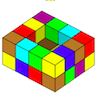Painting Possibilities

Age 7 to 11 Challenge Level:

This task, written for the National Young Mathematicians' Award 2016, involves open-topped boxes made with interlocking cubes. Explore the number of units of paint that are needed to cover the boxes. . . .Being Resourceful - Primary Number

Age 5 to 11 Challenge Level:

Number problems at primary level that require careful consideration.Triangles All Around

Age 7 to 11 Challenge Level:

Can you find all the different triangles on these peg boards, and find their angles?Combining Cuisenaire

Age 7 to 11 Challenge Level:

Can you find all the different ways of lining up these Cuisenaire rods?Six Is the Sum

Age 7 to 11 Challenge Level:

What do the digits in the number fifteen add up to? How many other numbers have digits with the same total but no zeros?Fifteen Cards

Age 7 to 11 Challenge Level:

Can you use the information to find out which cards I have used?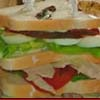Whose Sandwich?

Age 5 to 7 Challenge Level:

Chandra, Jane, Terry and Harry ordered their lunches from the sandwich shop. Use the information below to find out who ordered each sandwich.Growing Garlic

Age 5 to 7 Challenge Level:

Ben and his mum are planting garlic. Use the interactivity to help you find out how many cloves of garlic they might have had.Button-up

Age 5 to 7 Challenge Level:

My coat has three buttons. How many ways can you find to do up all the buttons?Teddy Town

Age 5 to 14 Challenge Level:

There are nine teddies in Teddy Town - three red, three blue and three yellow. There are also nine houses, three of each colour. Can you put them on the map of Teddy Town according to the rules?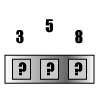Briefcase Lock

Age 5 to 7 Challenge Level:

My briefcase has a three-number combination lock, but I have forgotten the combination. I remember that there's a 3, a 5 and an 8. How many possible combinations are there to try?Maths Trails

Age 7 to 14

The NRICH team are always looking for new ways to engage teachers and pupils in problem solving. Here we explain the thinking behind maths trails.Making Trains

Age 5 to 7 Challenge Level:

Can you make a train the same length as Laura's but using three differently coloured rods? Is there only one way of doing it?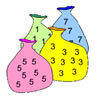Ordered Ways of Working Lower Primary

Age 5 to 7 Challenge Level:

These activities lend themselves to systematic working in the sense that it helps to have an ordered approach.More Children and Plants

Age 7 to 14 Challenge Level:

This challenge extends the Plants investigation so now four or more children are involved.More Plant Spaces

Age 7 to 14 Challenge Level:

This challenging activity involves finding different ways to distribute fifteen items among four sets, when the sets must include three, four, five and six items.Same Length Trains

Age 5 to 7 Challenge Level:

How many trains can you make which are the same length as Matt's, using rods that are identical?Multiples Grid

Age 7 to 11 Challenge Level:

What do the numbers shaded in blue on this hundred square have in common? What do you notice about the pink numbers? How about the shaded numbers in the other squares?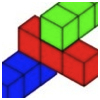Building with Longer Rods

Age 7 to 14 Challenge Level:

A challenging activity focusing on finding all possible ways of stacking rods.Three Ball Line Up

Age 5 to 7 Challenge Level:

Use the interactivity to help get a feel for this problem and to find out all the possible ways the balls could land.A Mixed-up Clock

Age 7 to 11 Challenge Level:

There is a clock-face where the numbers have become all mixed up. Can you find out where all the numbers have got to from these ten statements?Reach 100

Age 7 to 14 Challenge Level:

Choose four different digits from 1-9 and put one in each box so that the resulting four two-digit numbers add to a total of 100.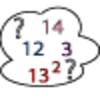Seven Square Numbers

Age 7 to 11 Challenge Level:

Add the sum of the squares of four numbers between 10 and 20 to the sum of the squares of three numbers less than 6 to make the square of another, larger, number.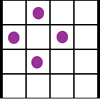Square Corners

Age 7 to 11 Challenge Level:

What is the greatest number of counters you can place on the grid below without four of them lying at the corners of a square?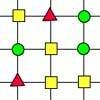A Shapely Network

Age 7 to 11 Challenge Level:

Your challenge is to find the longest way through the network following this rule. You can start and finish anywhere, and with any shape, as long as you follow the correct order.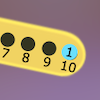Are You Well Balanced?

Age 5 to 7 Challenge Level:

Can you work out how to balance this equaliser? You can put more than one weight on a hook.Mystery Matrix

Age 7 to 11 Challenge Level:

Can you fill in this table square? The numbers 2 -12 were used to generate it with just one number used twice.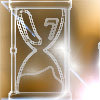Two Egg Timers

Age 7 to 11 Challenge Level:

You have two egg timers. One takes 4 minutes exactly to empty and the other takes 7 minutes. What times in whole minutes can you measure and how?Making Maths: Double-sided Magic Square

Age 7 to 14 Challenge Level:

Make your own double-sided magic square. But can you complete both sides once you've made the pieces?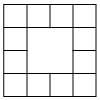Prison Cells

Age 7 to 11 Challenge Level:

There are 78 prisoners in a square cell block of twelve cells. The clever prison warder arranged them so there were 25 along each wall of the prison block. How did he do it?A-magical Number Maze

Age 7 to 11 Challenge Level:

This magic square has operations written in it, to make it into a maze. Start wherever you like, go through every cell and go out a total of 15!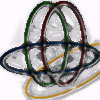Bean Bags for Bernard's Bag

Age 7 to 11 Challenge Level:

How could you put eight beanbags in the hoops so that there are four in the blue hoop, five in the red and six in the yellow? Can you find all the ways of doing this?